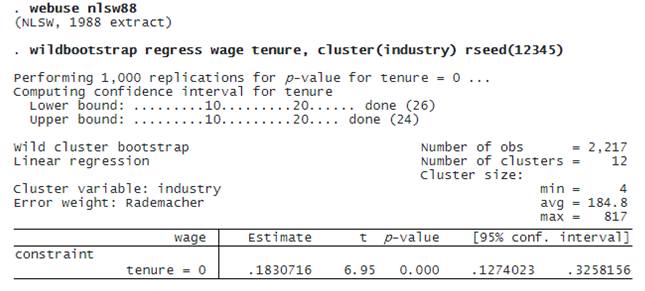WILD CLUSTER BOOTSTRAP

OVERVIEW

The WCB proposed by Cameron, Gelbach, and Miller (2008), provides an alternative to the cluster–robust variance estimator when you have either a small number of clusters or an uneven number of observations across clusters.

When we fit models with clustered observations, we often use a cluster–robust variance estimator, which relaxes the independence assumption for observations within each cluster. This estimator works well if we have many clusters and if the clusters do not differ too much in their numbers of observations. However, if this is not the case, we may obtain better estimates using the WCB.

Stata’s new wildbootstrap command estimates WCB p-values and confidence intervals (CIs) for tests of simple and composite linear hypotheses about parameters from linear regression models. These statistics can be obtained when fitting linear regression models such as those fit with regress, models with a large indicator-variable set such as those fit with areg, and fixed-effects models such as those fit with xtreg, fe.

WILD CLUSTER BOOTSTRAP IN ACTION!

We would like to see the effect of tenure on wages and to account for clusters at the industry level. Here we use a wage dataset from 1988 with only 12 clusters with substantially varying cluster sizes, from 4 to 817, deviating from the assumptions required for the cluster–robust variance estimator to be reliable.

We fit a linear regression and compute WCB statistics for a test that the coefficient on tenure is zero. We set the seed using rseed() for reproducibility.The estimated coefficient on tenure is 0.183. The equal-tailed p-value for the test that the coefficient equals zero is less than 0.001; the confidence interval is [0.127, 0.326].

Here we used the default Rademacher weights used for the sampling algorithm of the wild bootstrap. Mammen, Webb, gamma, and normal weights are also available.

While this example is simple, wildbootstrap is quite flexible. You can fit models with many covariates; compute WCB statistics for some or all of them. You can even specify a hypothesis involving multiple coefficients. If, for instance, you wish to test that coefficients on x1 and x2 are equal, add the test(x1=x2) option to your wildbootstrap command.